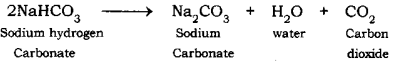# (i) Name the products formed when sodium hydrogen carbonate is heated

(i) Name the products formed when sodium hydrogen carbonate is heated.
(ii) Write the chemical equation for the reaction involved in the above.

(i) When sodium hydrogen carbonate is heated, sodium carbonate is heated, sodium carbonate (NO2CO3), water (H2O), and carbon dioxide (CO2) gases are formed.

(ii) The chemical equation involved in the above reaction can be represented as: